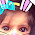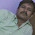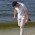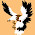Monday, May 31, 2010

Excel Formula : Calculating Age

Today I am sharing with a long formula for calculating age based in Date of Birth as input.

=YEAR(DATE(YEAR(TODAY())-YEAR(A1),MONTH(TODAY())-MONTH(A1)+1,DAY(TODAY())-DAY(A1)+1))-1900&" Years "&IF(MONTH(DATE(YEAR(TODAY())-YEAR(A1),MONTH(TODAY())-MONTH(A1)+1,DAY(TODAY())-DAY(A1)+1))-1=0,"",MONTH(DATE(YEAR(TODAY())-YEAR(A1),MONTH(TODAY())-MONTH(A1)+1,DAY(TODAY())-DAY(A1)+1))-1&" Months ")&IF(DAY(DATE(YEAR(TODAY())-YEAR(A1),MONTH(TODAY())-MONTH(A1)+1,DAY(TODAY())-DAY(A1)+1))-1=0,"",DAY(DATE(YEAR(TODAY())-YEAR(A1),MONTH(TODAY())-MONTH(A1)+1,DAY(TODAY())-DAY(A1)+1))-1&" Days")

This formula calculates age of a person with the accuracy of exact years , months and days

This considers that the Date of Birth is available at Cell A1 of the worksheet. You will need to replace this

Download excel file containing formula to calculate age based on Date of Birth

1.A shorter formula:

=CONCATENATE(DATEDIF(A1,TODAY(),"y")," years ",MOD(DATEDIF(A1,TODAY(),"m"),12), " Months")

2.jialin: pretty good, but don't think DATEDIF is in Excel 2007. It was in earlier versions, right? Perhaps something similar can be accomplished with DAYS360?

I was thinking something along these lines:
=TEXT(TODAY()-A1,"YYYY")-1900&" Years "&MONTH(TODAY()-A1)-1&" Months "&DAY(TODAY()-A1)&" Days"

Still, all of our formulas needed to be modified to include logic for singular values and zero values for all three: years, months, and days.

3.Hello Mr Gupta

Following is not very short but comes close to figure out the remaining nights sleep till the next birthday. Here C1=date today b2 = date of birth and d2 = calculated birthday this year
=IF(MONTH(\$C\$1)<MONTH(B2);"still "&(DATEVALUE(D2))-TODAY()&" nights sleep";IF(AND(MONTH(\$C\$1)=MONTH(B2);DAY(\$C\$1)<DAY(B2));"still "&(DATEVALUE(D2))-TODAY()&" night(s) sleep";IF(AND(MONTH(\$C\$1)=MONTH(B2);DAY(\$C\$1)=DAY(B2));"**Heep Heep Huray !!!!**";"still "&EDATE(Datevalue(D2);12)-TODAY()&" nights sleep")))

4.@Squash DATEDIF works in 2003 and 2007 as well. It is just that it is not a regular excel function. It is a VBA function and hence there is no help available in excel and the argument directions don't turn up.

5.Hello Yogesh ji,

I need a help from you.

I want to put a formula in sheet2 which should calculate as per the last (filled) row of sheet1.

for eg.
in sheet1 Cell A61 is 408 & B61 is 203... I want to show the sum of these two in the Cell A12 of sheet2.. but I want the firmula to use the lastest row of Sheet1... say if A62 is 410 & B62 is 200 then A12 of sheet2 should show "610" and like wise everytime there is new entry in sheet1.

hope I am clear in my question.

Thanks

6.I'm not sure why you posted this particular question under this blog article. However, assuming there are **no** blank cells in Column A (which I'm assuming will always have the last value on the sheet in it), then try this formula...

=LOOKUP(2,1/(A1:A65535<>""),A:A)+INDEX(B2:B10,COUNTA(A:A))

Rick Rothstein (MVP - Excel)

7.Hi Amit

You can use following formula to get the last entered numeric value in column A of sheet1

=INDEX(Sheet1!A:A,MATCH(9.99999999999999E+307,Sheet1!A:A))

I hope you will be able to modify this to get value in column B

Regards//Yogesh Gupta

8.Hello Yogesh ji,

thanks for the formula, its working.

@Rick,

thanks

9.hi yogesji, i have an excel sheet having multiple data and every data row have a blank row beetween two rows. i want to delete the blank row in one shot, is it possible pls. help me.

10.Hai Sir, you done great job for intermediate learner for excel we want to some accounting templates like daybook, ledger and balance sheet:
once we enter the transaction that can automatically updated in ledger and balance sheet please send me my mail id is razaravi@gmail.com

11.This comment has been removed by the author.

12.Dear SIr,
can we calculate age by the following formula. Please answer me. (put DOB at Cell A1)

=+DATEDIF(A1,NOW(),"Y")&" Years, "&DATEDIF(A1,NOW(),"ym")&" Months, "&DATEDIF(A1,NOW(),"md")&" Day."

From: Sujit Dhar
sujit_dhar1025@hotmail.com

13.Try This ;)

=DATEDIF(A1,TODAY(),"y") & " years " & DATEDIF(A1,TODAY(),"ym") & " months " & DATEDIF(A1,TODAY(),"md")+1 & " days "

14.Sir,

My self santosh I want to some information about my excel sheet simplifying.

15.sir, my self anoop singh. I want to pint a check book in excel work sheet, but i hve sme prblm like i want to print numeric value in text. Supose 120460.But spellcurr()formula RETURN value one hudred twnty thousnd four hundrd sixty only. But I want print "One lack twenty thounsd four hundrd sixty only"
I hope Sir u help me better.

16.I'm using the =INT((TODAY()-AB2)/365.25). It does give me the age, but if I don't have a date in the birthday cell(AB2) I get a "111" in the age cell.
How can I make the age cell blank if there is no date in the birthday cell? Thanks!

17.hi there
i recently created a worksheet for paid and unpaid invoices and just need to know if i can somehow put a tick and a cross sign showing whether it has been paid or not.
Regards,
Shishir Sharma

18.@gfizz....if(A! <> "",int((today()-A1)365.25),"no date")

this is just if operator logic...if u find other logic plz let me know

19.=DATEDIF(Bd,TODAY(),"y") & " Years "&DATEDIF(Bd,TODAY(),"ym") & " Months "&DATEDIF(Bd,TODAY(),"md") &" Days"

20.hello, i need to know how to convert word to number and number to word in excel 2007. i've seen your spellcurr formula but i don't have it.

21.i wanto view to oracle data .dat file in excle sheet.

22.Yogesh ji please help me: How can i convert Date into Text format i.e. 17-08-1977 as Seventeenth August Nineteen Seventy Seven.. plz

23.Dear sir,

I update in excel of our Sales order, when its gone out I put Date of dispatch,

I want when I put date, it should be, Hide in the next sheet, only show pending order which is not gone.

if its possible please inform me sir,

24.Dear Sir,

Can we have the formula for tracking the change in number of two cloumns by auto change in the cell color ( +ve, -ve and equal values)

25.nice information i found online date of birth calculator http://www.onlinecalculator1.com/Date-of-birth-calculator.php

26.nice information i found online date of birth calculator http://www.onlinecalculator1.com/Date-of-birth-calculator.php

27.In the event that you on the lookout for means of just how exactly to learn how old someone's afterward you definitely may discover ideas you may take to this. To recover such info you want to consider about most of the spots where folks supply their arrival information along with also these places needs to be reachable during internet hunts. You ought to decide to try age calculator by that you are able to estimate your era rapidly.I highly suggested you this age calculator through which you can calculate your age in Years, months, Days, weeks, hours, minutes and seconds.

28.If you are Windows customer and a music fan, you have definitely been influenced to get Garageband for Windows 10 and Garageband is one of the favored music creating an app.

29.Find your age with the assistance of particular handy age calculator. You can readily calculate age in years, months, days, weeks, hours, and minutes together with the assistance of the age calculator.

30.Di bawah ini ialah point poin yang akan menuturkan mengenai begitu menyenangkan serta menguntungkannya bermain di situs poker online cukup dengan modal Rp 10rb saja
asikqq
dewaqq
sumoqq
interqq
pionpoker
bandar ceme terpercaya
hobiqq
paito warna
forum prediksi

31.Shalom, Om swastiastu, Namo buddhaya, Salam kebajikan, pada artikel kali ini kami akan memberikan kepada kamu Panduan Cara Bet 2D Togel Klik4D Online yang tersedia di S128cash. Berhubung permainan togel adalah game legendaris di Indonesia, tahukah kamu kalau game togel sekarang bisa dimainkan secara online ? (Baca Selengkapnya Disini...)